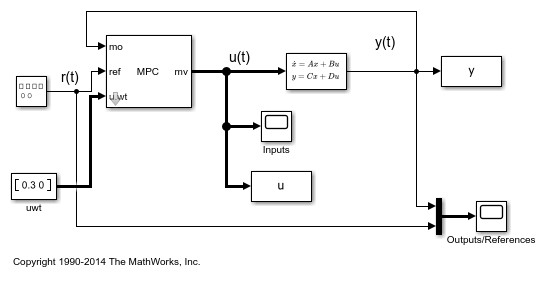Main Content

# Setting Targets for Manipulated Variables

This example shows how to design a model predictive controller for a plant with two inputs and one output with target setpoint for a manipulated variable.

### Define Plant Model

The linear plant model has two inputs and two outputs.

```N1 = [3 1]; D1 = [1 2*.3 1]; N2 = [2 1]; D2 = [1 2*.5 1]; plant = ss(tf({N1,N2},{D1,D2})); A = plant.A; B = plant.B; C = plant.C; D = plant.D; x0 = [0 0 0 0]'; ```

### Design MPC Controller

Create MPC controller.

```Ts = 0.4; % Sample time mpcobj = mpc(plant,Ts,20,5); ```
```-->The "Weights.ManipulatedVariables" property of "mpc" object is empty. Assuming default 0.00000. -->The "Weights.ManipulatedVariablesRate" property of "mpc" object is empty. Assuming default 0.10000. -->The "Weights.OutputVariables" property of "mpc" object is empty. Assuming default 1.00000. ```

Specify weights.

```mpcobj.weights.manipulated = [0.3 0]; % weight difference MV#1 - Target#1 mpcobj.weights.manipulatedrate = [0 0]; mpcobj.weights.output = 1; ```

Define input specifications.

```mpcobj.MV = struct('RateMin',{-0.5;-0.5},'RateMax',{0.5;0.5}); ```

Specify target setpoint `u = 2` for the first manipulated variable.

```mpcobj.MV(1).Target=2; ```

### Simulation Using Simulink®

To run this example, Simulink® is required.

```if ~mpcchecktoolboxinstalled('simulink') disp('Simulink(R) is required to run this example.') return end ```

Simulate.

```mdl = 'mpc_utarget'; open_system(mdl) % Open Simulink(R) Model sim(mdl); % Start Simulation ```
```-->Converting model to discrete time. -->Assuming output disturbance added to measured output channel #1 is integrated white noise. -->The "Model.Noise" property of the "mpc" object is empty. Assuming white noise on each measured output channel. ``````bdclose(mdl) ```

## Support

#### Implementing an Adaptive Cruise Controller with Simulink

Download technical paper